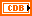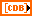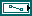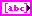# Comparison of MathScript RT Module and the MATLAB® Software Data Types in LabVIEW

## LabVIEW 2018 Help

Edition Date: March 2018
Part Number: 371361R-01
View Product InfoLabVIEW 2016 HelpLabVIEW 2017 HelpLabVIEW 2018 HelpLabVIEW 2019 HelpLabVIEW 2020 Help

The following table shows LabVIEW data types and the corresponding data types in the MATLAB® software. (MathScript RT Module) This table also shows the corresponding LabVIEW MathScript data types. You can change the data type of an input or output terminal on a script node.

LabVIEW Data Type MATLAB® Script Node Data Type MathScript Node Data TypeDouble-precision, floating-point numeric
Real Scalar»DBLComplex double-precision, floating-point numeric
Complex Scalar»CDB1D array of double-precision, floating-point numeric
1-D Array of Real 1D-Array»DBL 1D1D array of complex double-precision, floating-point numeric
1-D Array of Complex 1D-Array»CDB 1DMultidimensional array of double-precision, floating-point numeric
2-D Array of Real Matrix»Real Matrix (2D only)Multidimensional array of complex double-precision, floating-point numeric
2-D Array of Complex Matrix»Complex Matrix (2D only)String
String Scalar»StringPath
Path N/A1D array of string
N/A 1D-Array»String 1D

(MathScript RT Module) You can use additional data types with the MathScript Node, including the state-space, transfer function, and zero-pole-gain models of the LabVIEW Control Design and Simulation Module. You must have the Control Design and Simulation Module installed to use these models. Refer to the Control Design and Simulation Module documentation for more information about these models.Note  MathScript represents strings internally as arrays of the corresponding ASCII character codes.

The MATLAB script node and the MathScript Node handle 1D array inputs only by row. To change the orientation of a 1D array from row to column or column to row, transpose the array before you perform operations on elements of the array.

Use the Conversion VIs and functions or the Path/Array/String Conversion functions to convert a LabVIEW data type to a data type that the MathScript RT Module or the MATLAB software supports.